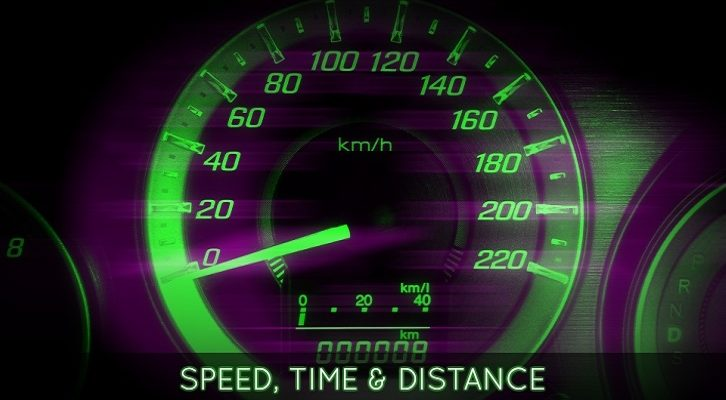# Races – Two Questions

Have given below two questions on races. For a recap of the basics on Races, see questions here and the solutions here.

1. Two friends A and B simultaneously start running around a circular track . They run in the same direction. A travels at 6m/s and B runs at b m/s. If they cross each other at exactly two points on the circular track and b is a natural number less than 30, how many values can b take?

2. Three friends A, B and C decide to run around a circular track. They start at the same time and run in the same direction. A is the quickest and when A finishes a lap, it is seen that C is as much behind B as B is behind A. When A completes 3 laps, C is the exact same position on the circular track as B was when A finished 1 lap. Find the ratio of the speeds of A,B and C?

## One comment

1.Neha Advani says:

ans 1 is 6?